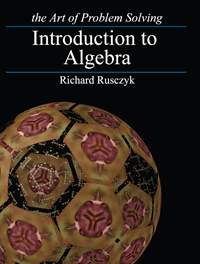# AoPS Introduction to Algebra Text and Solution Set

\$ 67.00
Paperback
Text: 656 pages. Solutions: 312 pages.
A thorough introduction for students in grades 6-9 to algebra topics such as linear equations, ratios, quadratic equations, special factorizations, complex numbers, graphing linear and quadratic equations, linear and quadratic inequalities, functions, polynomials, exponents and logarithms, absolute value, sequences and series, and more!

### Overview

Learn the basics of algebra from former USA Mathematical Olympiad winner and Art of Problem Solving founder Richard Rusczyk. Topics covered in the book include linear equations, ratios, quadratic equations, special factorizations, complex numbers, graphing linear and quadratic equations, linear and quadratic inequalities, functions, polynomials, exponents and logarithms, absolute value, sequences and series, and much more!

The text is structured to inspire the reader to explore and develop new ideas. Each section starts with problems, giving the student a chance to solve them without help before proceeding. The text then includes solutions to these problems, through which algebraic techniques are taught. Important facts and powerful problem solving approaches are highlighted throughout the text. In addition to the instructional material, the book contains well over 1000 problems. The solutions manual contains full solutions to all of the problems, not just answers.

This book can serve as a complete Algebra I course, and also includes many concepts covered in Algebra II. Middle school students preparing for MATHCOUNTS, high school students preparing for the AMC, and other students seeking to master the fundamentals of algebra will find this book an instrumental part of their mathematics libraries.

Table of Content

Sample Pages

Text ISBN: 978-1-934124-14-7
Solutions ISBN: 978-1-934124-15-4